Calculus Course Baseline Rendering Baseline vs Progressive Rendering

• Slides: 45Calculus CourseBaseline Rendering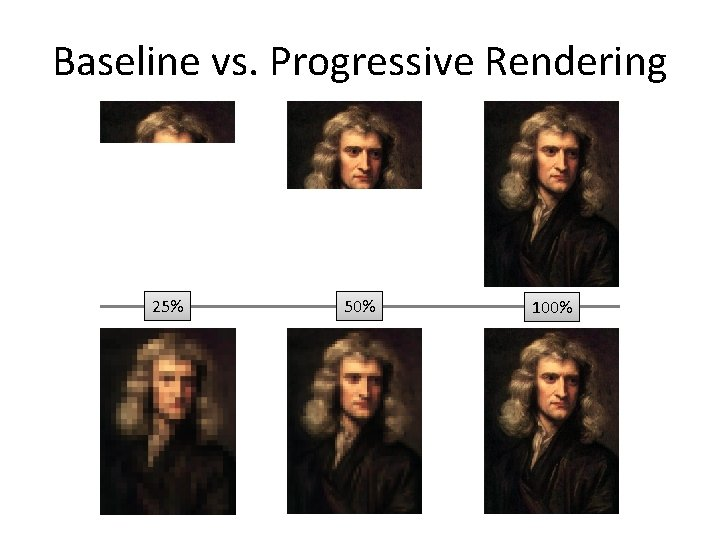Baseline vs. Progressive Rendering 25% 50% 100%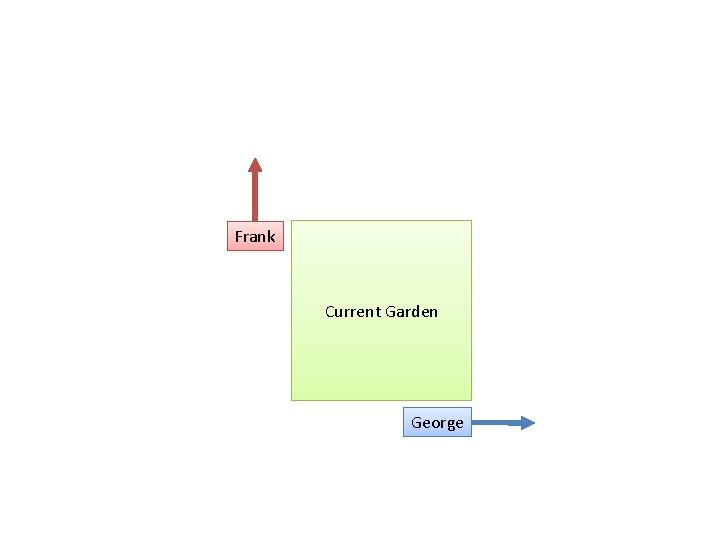Frank Current Garden George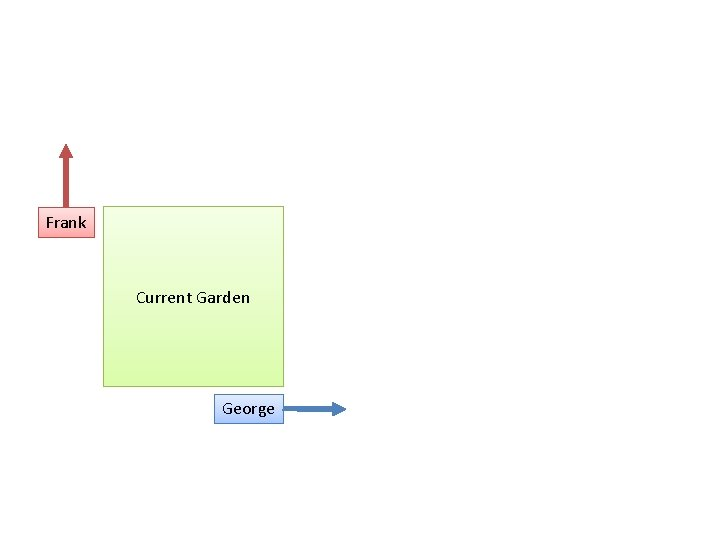Frank Current Garden GeorgeFrank Current Garden George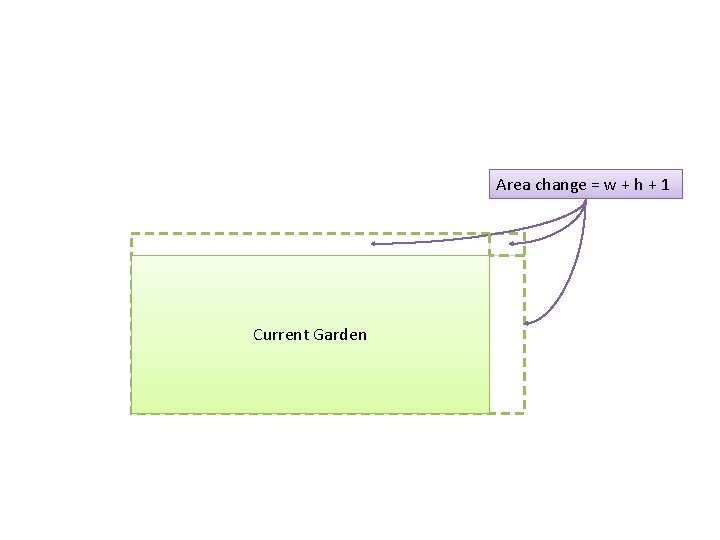Area change = w + h + 1 Current GardenSystem Derivative A+B+C []+[]+[] A*B*C []+[]+[] A^(B^C) []+[]+[] A^B / C []+[]+[] Three inputs, 3 changing perspectives to includeScenario With 3 Parts Change Simplifies To A + B + C A * B * C A ^ B ^ C A * B / … C A’s changes + B’s changes + C’s changes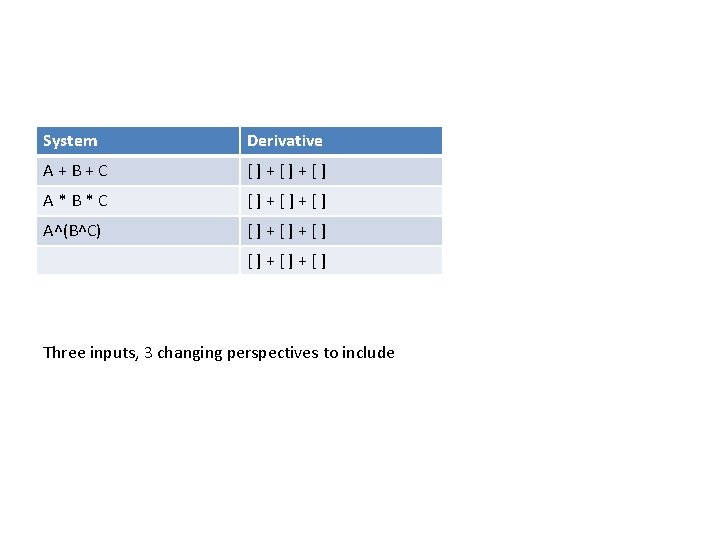System Derivative A+B+C []+[]+[] A*B*C []+[]+[] A^(B^C) []+[]+[] Three inputs, 3 changing perspectives to includeScenario With 2 Parts System Derivative A + B A*B*C []+[]+[] A^(B^C) []+[]+[] … Fuzzy Viewpoint Fuzzy Derivati A’s ve changes + B’s changesCourse Strategy/Content • Lesson 1: Intro • Lesson 2: Calculus In a Few Short MinutesLesson 4: Splitting a cake – Goal: Another scenario to examine step-by-step – You’re having a birthday part. About to cut the cake. • Friend comes in • And another – How do you model the changes? How much cake are you losing with each additional person? – Inverse relationship (your share is 1/x). How is your share changing as x (the number of people) increases? – So… can you see how ¼ = 1 [starting] – ½ [one person] - 1/6 [another comes in] – And 1/5 = 1 – ½ - 1/6 – 1/12 [and another] – Neat! – How many people does it take to save \$50/person? (1/20 the total? ) – Calculus. Transaction-by-transcation. • You could manually start adding differences… but calculus gave you the pattern! • Where do you need to be to see the change you need ? – Would have noticed that pattern on your own?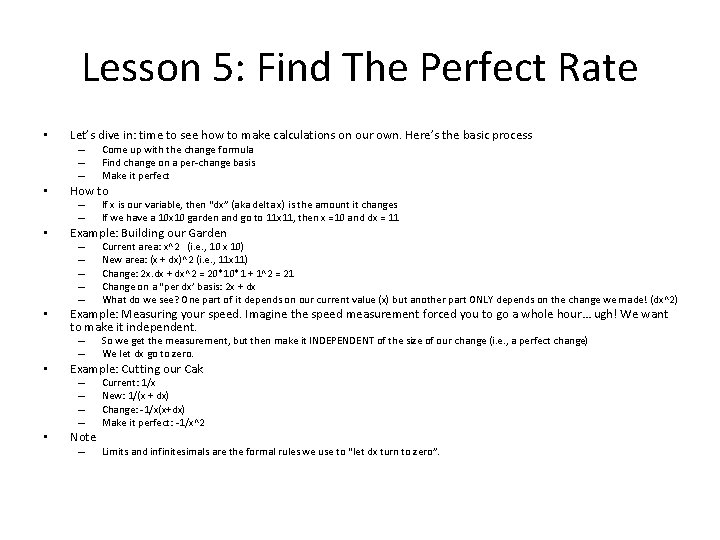Lesson 5: Find The Perfect Rate • Let’s dive in: time to see how to make calculations on our own. Here’s the basic process – – – • How to – – • So we get the measurement, but then make it INDEPENDENT of the size of our change (i. e. , a perfect change) We let dx go to zero. Example: Cutting our Cak – – • Current area: x^2 (i. e. , 10 x 10) New area: (x + dx)^2 (i. e. , 11 x 11) Change: 2 x. dx + dx^2 = 20*10*1 + 1^2 = 21 Change on a “per dx’ basis: 2 x + dx What do we see? One part of it depends on our current value (x) but another part ONLY depends on the change we made! (dx^2) Example: Measuring your speed. Imagine the speed measurement forced you to go a whole hour… ugh! We want to make it independent. – – • If x is our variable, then “dx” (aka delta x) is the amount it changes If we have a 10 x 10 garden and go to 11 x 11, then x =10 and dx = 11 Example: Building our Garden – – – • Come up with the change formula Find change on a per-change basis Make it perfect Note – Current: 1/x New: 1/(x + dx) Change: -1/x(x+dx) Make it perfect: -1/x^2 Limits and infinitesimals are the formal rules we use to “let dx turn to zero”.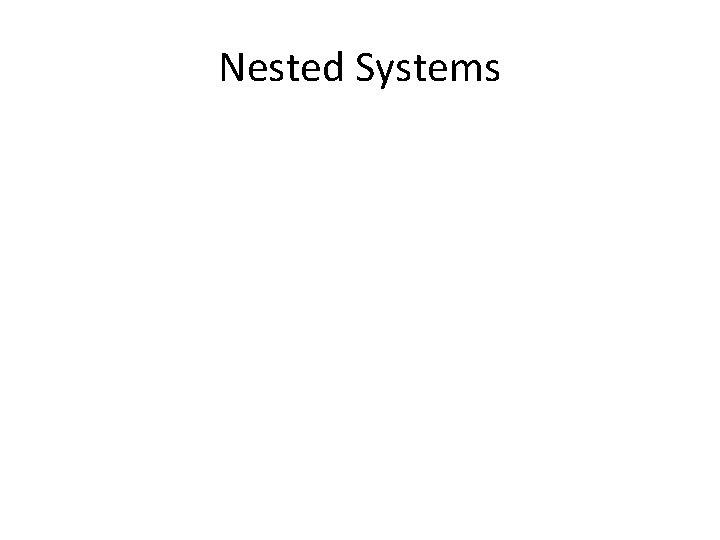Nested Systems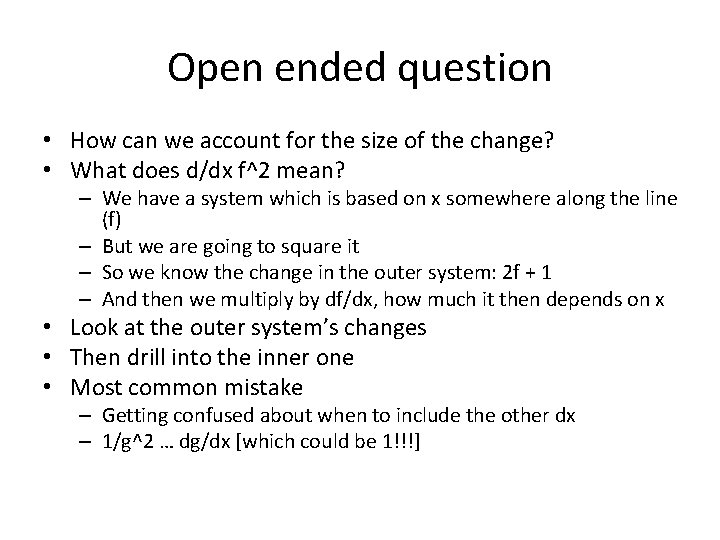Open ended question • How can we account for the size of the change? • What does d/dx f^2 mean? – We have a system which is based on x somewhere along the line (f) – But we are going to square it – So we know the change in the outer system: 2 f + 1 – And then we multiply by df/dx, how much it then depends on x • Look at the outer system’s changes • Then drill into the inner one • Most common mistake – Getting confused about when to include the other dx – 1/g^2 … dg/dx [which could be 1!!!]Lesson 6: Exchanging Currency • • Pretend you can get \$3 in profit per square foot Make a clap… how much more profit will you get? – – • We have a chain reaction We clap, which makes area go up… Which makes profit go up… Which makes EUR go up And later – Chain reaction. Change A (how much does it change? ) which changes B (how much does it change? ) which changes C (how much does it change? ), on and on. • We have a 2 x 2 and we clap… – Which makes our area go up 5 feet (2 x + 1) – Which makes our profit go up (3) – Which could be converted • Now, let’s say our profit was not just linear… another proportion? – Clap, which is 2 x + 1 – And that is how long we run another clapper for (2 x + 1) over there!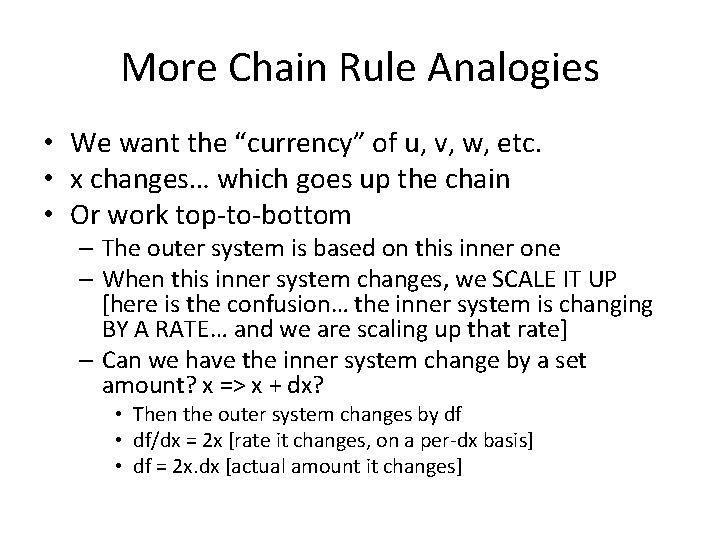More Chain Rule Analogies • We want the “currency” of u, v, w, etc. • x changes… which goes up the chain • Or work top-to-bottom – The outer system is based on this inner one – When this inner system changes, we SCALE IT UP [here is the confusion… the inner system is changing BY A RATE… and we are scaling up that rate] – Can we have the inner system change by a set amount? x => x + dx? • Then the outer system changes by df • df/dx = 2 x [rate it changes, on a per-dx basis] • df = 2 x. dx [actual amount it changes]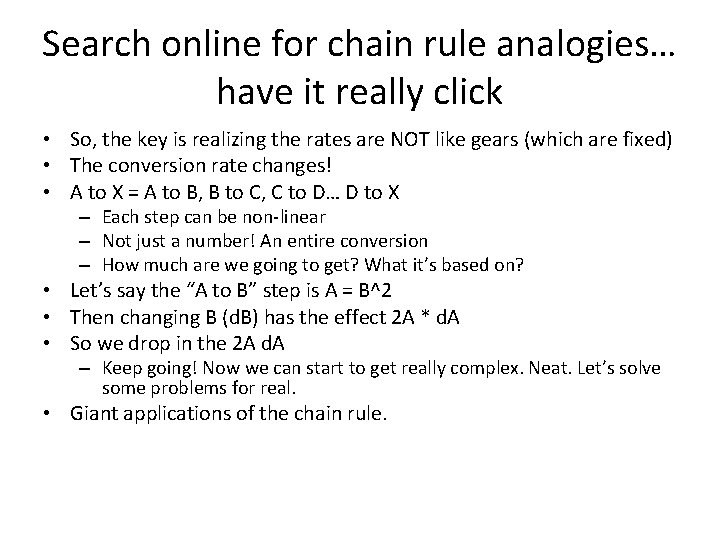Search online for chain rule analogies… have it really click • So, the key is realizing the rates are NOT like gears (which are fixed) • The conversion rate changes! • A to X = A to B, B to C, C to D… D to X – Each step can be non-linear – Not just a number! An entire conversion – How much are we going to get? What it’s based on? • Let’s say the “A to B” step is A = B^2 • Then changing B (d. B) has the effect 2 A * d. A • So we drop in the 2 A d. A – Keep going! Now we can start to get really complex. Neat. Let’s solve some problems for real. • Giant applications of the chain rule.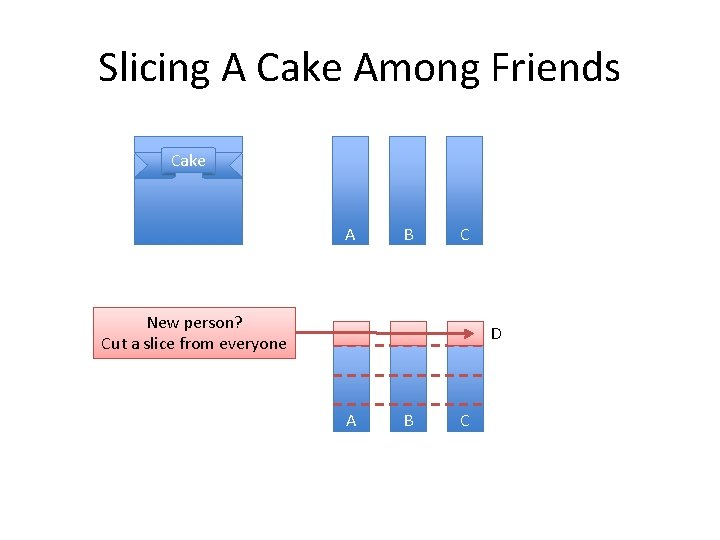Slicing A Cake Among Friends Cake A B C New person? Cut a slice from everyone D A B C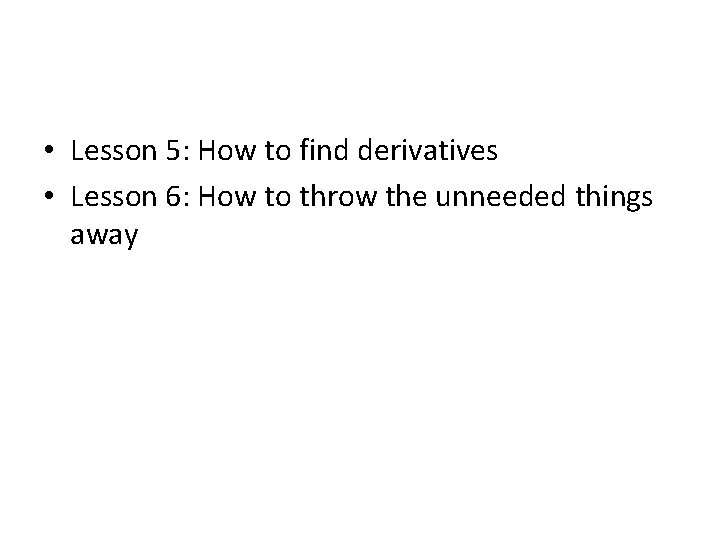• Lesson 5: How to find derivatives • Lesson 6: How to throw the unneeded things awayAnalogy Step-by-Step Thinking Banking Track your bank balance. Derivative is the change, apply all previous transactions to get your balance Sports Track the game’s score. Derivative is the play-by-play, apply all previous plays to get the current result Physics Track distance moved. Speed is the instant-by-second change, account for the speed each second to get total distance moved. Economics Track your profit. Marginal profit is the extra profit from producing 1 more widget (which may lower price). Total profit is accounting for the profit contribution from every widget made.Concept Goals • Want you to think step-by-step – Not just final results • Learn how to make better estimates – Go from discrete (large, noticeable steps) to continuous (perfectly smooth, undetectable steps) • Learn how to calculate the actual changes – Derivative: see what the next step will be – Integral • Mechanical: accumulate previous steps to get a total • Artistic: Figure out what shape the total is forming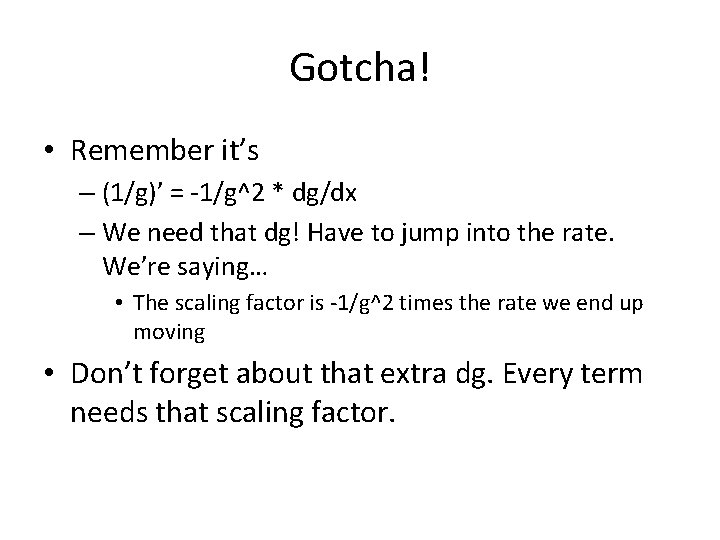Gotcha! • Remember it’s – (1/g)’ = -1/g^2 * dg/dx – We need that dg! Have to jump into the rate. We’re saying… • The scaling factor is -1/g^2 times the rate we end up moving • Don’t forget about that extra dg. Every term needs that scaling factor.X-Ray Original Rings Slices BoardsRing By Ring Timelapse 25% 50% 75% 100%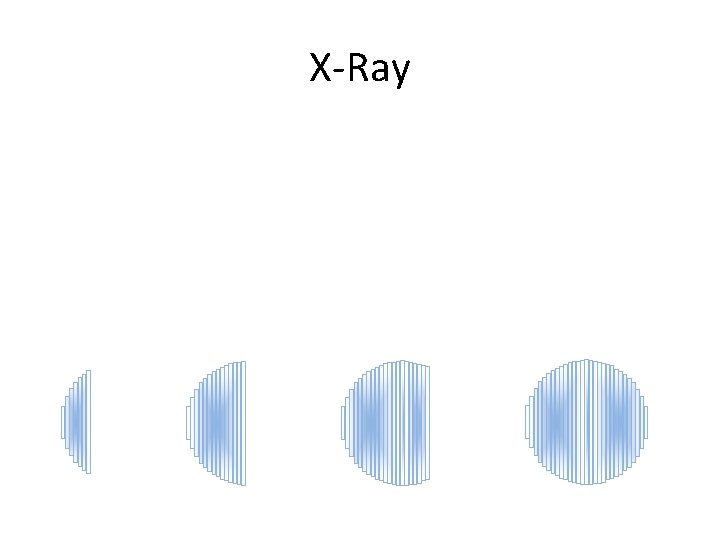X-Ray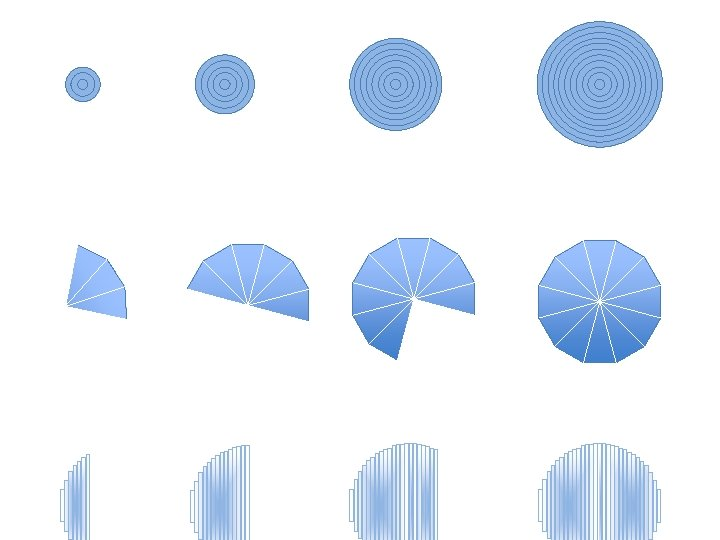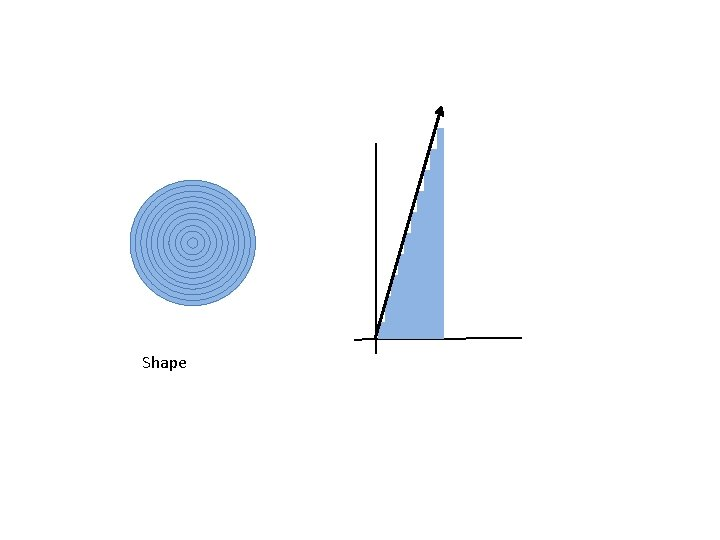Shape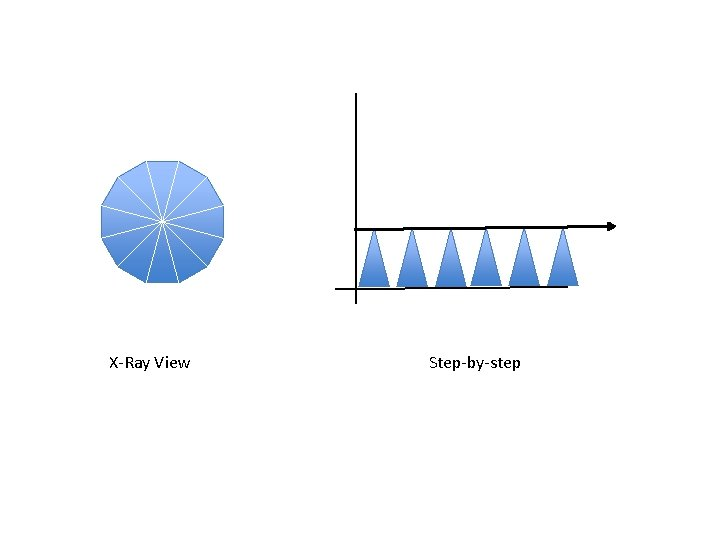X-Ray View Step-by-stepX-Ray Shape Step-by-stepX-Ray Strategy Ring-by-ring Slice-by-slice Board-by-board Visualization Step-by-Step Pattern Calculus Lingo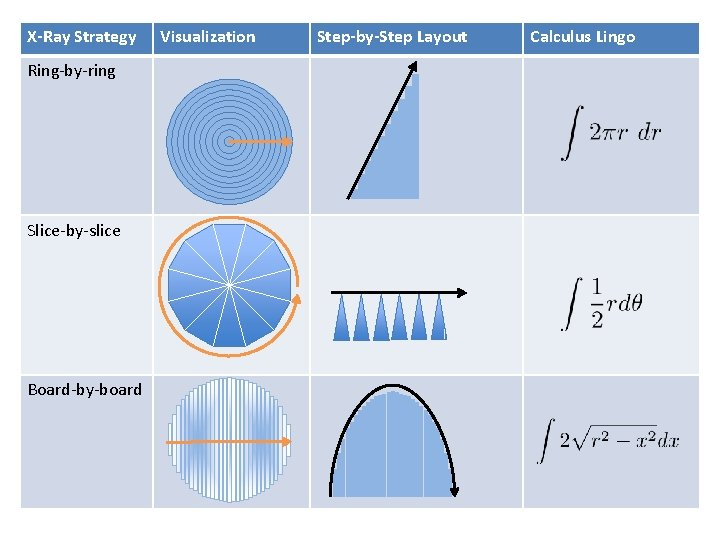X-Ray Strategy Ring-by-ring Slice-by-slice Board-by-board Visualization Step-by-Step Layout Calculus LingoX-Ray Strategy Ring-by-ring Slice-by-slice Board-by-board Visualization Step-by-Step Layout Calculus Lingo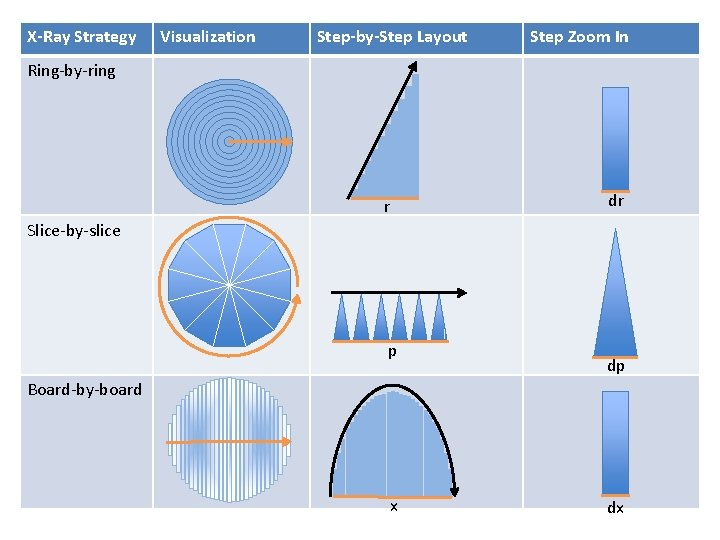X-Ray Strategy Visualization Step-by-Step Layout Step Zoom In Ring-by-ring r dr Slice-by-slice p dp Board-by-board x dxShape Animation Spin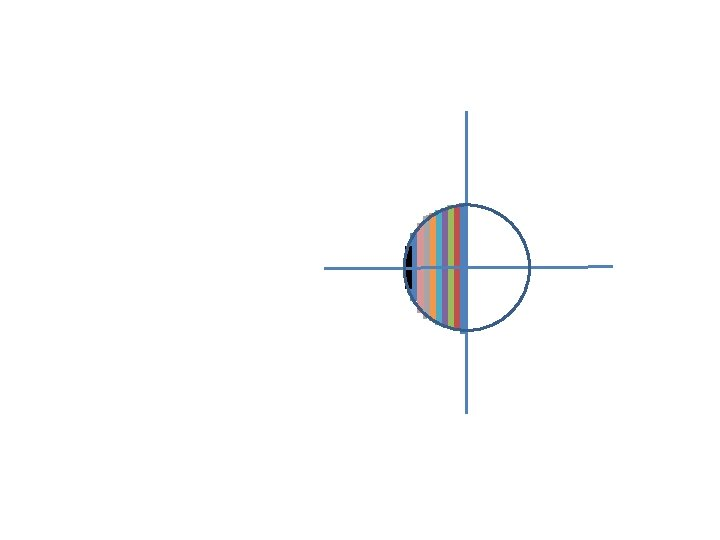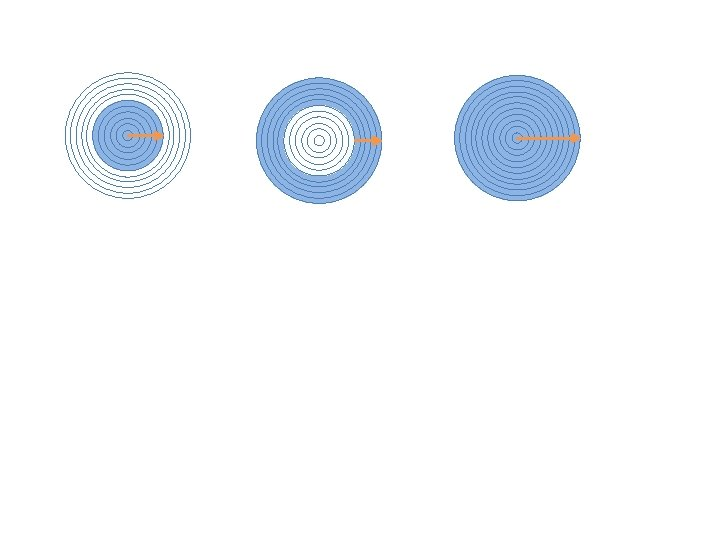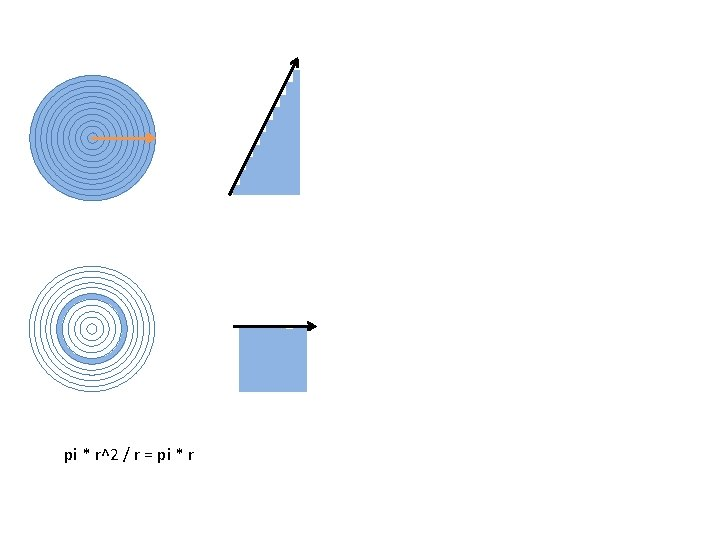pi * r^2 / r = pi * r